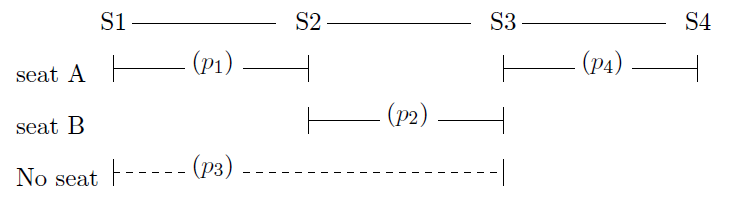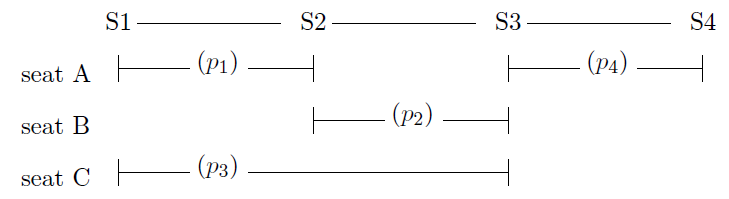Time Limit : sec, Memory Limit : KB
English

## Problem I Starting a Scenic Railroad Service

Jim, working for a railroad company, is responsible for planning a new tourist train service. He is sure that the train route along a scenic valley will arise a big boom, but not quite sure how big the boom will be.

A market survey was ordered and Jim has just received an estimated list of passengers' travel sections. Based on the list, he'd like to estimate the minimum number of train seats that meets the demand.

Providing as many seats as all of the passengers may cost unreasonably high. Assigning the same seat to more than one passenger without overlapping travel sections may lead to a great cost cutback.

Two different policies are considered on seat assignments. As the views from the train windows depend on the seat positions, it would be better if passengers can choose a seat. One possible policy (named policy-1') is to allow the passengers to choose an arbitrary seat among all the remaining seats when they make their reservations. As the order of reservations is unknown, all the possible orders must be considered on counting the required number of seats.

The other policy (named policy-2') does not allow the passengers to choose their seats; the seat assignments are decided by the railroad operator, not by the passengers, after all the reservations are completed. This policy may reduce the number of the required seats considerably.

Your task is to let Jim know how di erent these two policies are by providing him a program that computes the numbers of seats required under the two seat reservation policies. Let us consider a case where there are four stations, S1, S2, S3, and S4, and four expected passengers $p_1$, $p_2$, $p_3$, and $p_4$ with the travel list below.

passenger from to
$p_1$ S1 S2
$p_2$ S2 S3
$p_3$ S1 S3
$p_4$ S3 S4

The travel sections of $p_1$ and $p_2$ do not overlap, that of $p_3$ overlaps those of $p_1$ and $p_2$, and that of $p_4$ does not overlap those of any others.

Let's check if two seats would suffice under the policy-1. If $p_1$ books a seat first, either of the two seats can be chosen. If $p_2$ books second, as the travel section does not overlap that of $p_1$, the same seat can be booked, but the other seat may look more attractive to $p_2$. If $p_2$ reserves a seat different from that of $p_1$, there will remain no available seats for $p_3$ between S1 and S3 (Figure I.1).Figure I.1. With two seats

With three seats, $p_3$ can find a seat with any seat reservation combinations by $p_1$ and $p_2$. $p_4$ can also book a seat for there are no other passengers between S3 and S4 (Figure I.2).Figure I.2. With three seats

For this travel list, only three seats suffice considering all the possible reservation orders and seat preferences under the policy-1.

On the other hand, deciding the seat assignments after all the reservations are completed enables a tight assignment with only two seats under the policy-2 (Figure I.3).Figure I.3. Tight assignment to two seats

### Input

The input consists of a single test case of the following format.

$n$
$a_1$ $b_1$
...
$a_n$ $b_n$


Here, the first line has an integer $n$, the number of the passengers in the estimated list of passengers' travel sections ($1 \leq n \leq 200 000$). The stations are numbered starting from 1 in their order along the route. Each of the following $n$ lines describes the travel for each passenger by two integers, the boarding and the alighting station numbers, $a_i$ and $b_i$, respectively ($1 \leq a_i < b_i \leq 100 000$). Note that more than one passenger in the list may have the same boarding and alighting stations.

### Output

Two integers $s_1$ and $s_2$ should be output in a line in this order, separated by a space. $s_1$ and $s_2$ are the numbers of seats required under the policy-1 and -2, respectively.

### Sample Input 1

4
1 3
1 3
3 6
3 6


### Sample Output 1

2 2


### Sample Input 2

4
1 2
2 3
1 3
3 4


### Sample Output 2

3 2


### Sample Input 3

10
84 302
275 327
364 538
26 364
29 386
545 955
715 965
404 415
903 942
150 402


### Sample Output 3

6 5